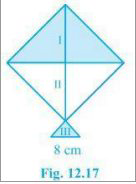# NCERT Solutions Class 9 Mathematics Solutions for Heron’s Formula - Exercise 12.2 in Chapter 12 - Heron’s Formula

A kite in the shape of a square with a diagonal 32 cm and an isosceles triangle of base 8 cm

and sides 6 cm each is to be made of three different shades as shown in Fig. 12.17. How much

paper of each shade has been used in it?As the kite is in the shape of a square, its area will be

\begin{array}{l} A=(1 / 2) \times(\text { diagonal })^{2} \\ \Rightarrow \text { Area of the kite }=(3 / 2) \times 32 \times 32=512 \mathrm{cm}^{2} \end{array}

\begin{aligned} &\Rightarrow 512 / 2 \mathrm{cm}^{2}=256 \mathrm{cm}^{2}\\ &\text { So, the total area of the paper that is required in each shade }=256 \mathrm{cm}^{2} \end{aligned}

For the triangle section (III),

The sides are given as 6 cm, 6 cm and 8 cm

Now, the semi perimeter of this isosceles triangle = (6 + 6 + 8)/2 cm = 10 cm

By using Heron's formula, the area of the III triangular piece will be

= √[s (s-a) (s-b) (s-c)]

\begin{array}{l} =\sqrt{10}(10-6)(10-6)(10-8) \mathrm{cm}^{2} \\ =\sqrt{10 \times 4 \times 4 \times 2 \mathrm{cm}^{2}} \\ =8 \mathrm{v} 6 \mathrm{cm}^{2} \end{array}

Related Questions

Lido

Courses

Teachers

Book a Demo with us

Syllabus

Maths
CBSE
Maths
ICSE
Science
CBSE

Science
ICSE
English
CBSE
English
ICSE
Coding

Terms & Policies

Selina Question Bank

Maths
Physics
Biology

Allied Question Bank

Chemistry
Connect with us on social media!